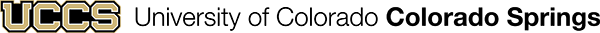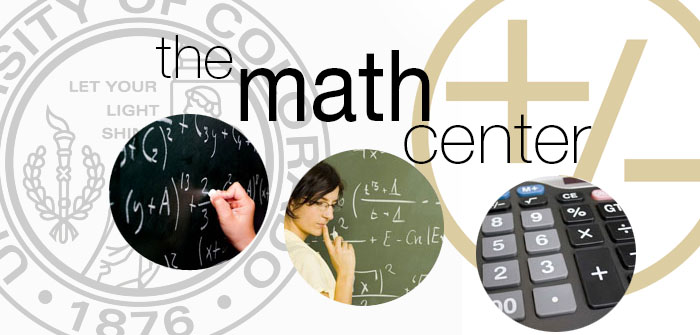# How do I Find Statistics?: Understanding Statistical Concepts

## Understand Statistical Concepts

Most courses about statistics introduce the concepts and applications of probability and statistical modeling, which include topics such as descriptive and inferential statistics. Within these topics are basic procedures and analysis tools that individuals working with statistics need to know. On this page, you will find information and resources to help you understand the following topics and concepts:

## Need More Help?The Mathematics Center offers drop-in tutoring and supplemental instruction in selected courses. They also have online and private tutoring available. The drop-in tutoring includes Social Science Statistics tutoring.

If you need more help or information about statistics, contact the Math Center at 719-255-3687, visit their website, or drop by and see them in Engineering 233.

## Reference Materials

Reference materials are located behind the Research Assistance desk on the 2nd floor of the Kraemer Family Library. Reference materials may not be checked out.

Inferential Statistics: statistics that allow one to draw conclusions or inferences from data. This means coming to conclusions about a population on the basis of data describing a sample.

Hypothesis Testing: The classical approach to assessing the statistical significance of findings. Basically, it involves comparing empirically observed sample findings with the theoretically expected finding that would occur if the null hypothesis were true.

p value: the probability of the observed value of the test statistic if the null hypothesis were true. When the p value is small (traditionally .05 or smaller), the researcher believes that the result was not due merely to chance.

Correlation: the extent to which two or more things or variables are related to one another. This is usually expressed as a correlation coefficient.

Correlation Coefficient: A number showing the degree to which two variables are related. Correlation coefficients range from -1.0 to +1.0. A correlation coefficient of 0 means that there is no relationship between the variables. The most common ways to compute correlation coefficients are:

Pearson Correlation Coefficient ( r ): a statistics showing the degree of linear relationship between two variables that have been measured on interval or ratio scales.

Spearman rho Correlation ( rs ): a statistic that shows the degree of monotonic relationship (a relationship in which an increase in one always produces an increase in the other) between two variables that are arranged in rank order (measured on an ordinal scale).

Regression: Techniques for establishing a regression equation. The equation indicates the nature and closeness of the relationship between two or more variables, specifically, the extent to which you can predict some by knowing other 0 the extent to which some are associated with others. The equation is often represented by a regression line (the straight line that comes closest to approximating a distribution of points in a scatter diagram).

Descriptive Statistics: procedures for summarizing, organizing, graphing, and, in general, describing quantitative data.

Measures of Central Tendency: Any of several statistics summaries that, in a single number, represent the typical or average number in a group of numbers. The measures include mean, median, and mode.

Mean: the average. To get the mean, you add up the values for each case and divide that sum by the total number of cases.

Median: the middle score or measurement in a set of ranked scores or measurements; the points that divides a distribution into two equal halves.

Mode: the most common number in a set of numbers.

Variance: a measure of the spread of scores in a distribution of scores, a measure of dispersion. The larger the variance, the father the individual cases are from the mean. The smaller the variance, the closer the individual scores are to the mean.

Standard Deviation: a measure of the average amount the scores in a distribution deviate from the mean. The standard deviation is the square root of the variance.

All definitions from: Vogt, W. P. & Johnson, R. B(2011). Dictionary of Statistics and Methodology: A Nontechnical Guide for the Social Sciences, (4th ed.) Los Angeles, CA: SAGE.

General Statistics Help